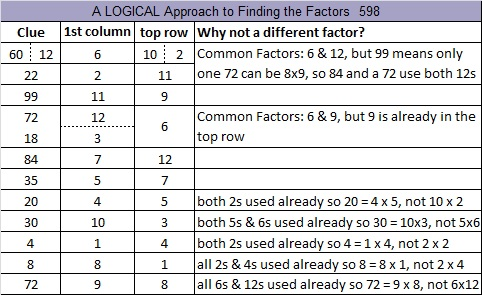# 598 and Level 6

598 is the sum of all the prime number from 17 to 71.

598 is also the hypotenuse of the Pythagorean triple 230-552-598. What is the greatest common factor of those three numbers?

Here’s another easy as 1-2-3 number fact from OEIS.org: (5^1) + (9^2) +(8^3) = 598.

Since 23 + 3 = 26 and 23 x 26 = 598, we know we are only 2 away from 24 x 25 = 600.Print the puzzles or type the solution on this excel file: 12 Factors 2015-08-24

—————————————————————————————————

• 598 is a composite number.
• Prime factorization: 598 = 2 x 13 x 23
• The exponents in the prime factorization are 1, 1, and 1. Adding one to each and multiplying we get (1 + 1)(1 + 1)(1 + 1) = 2 x 2 x 2 = 8. Therefore 598 has exactly 8 factors.
• Factors of 598: 1, 2, 13, 23, 26, 46, 299, 598
• Factor pairs: 598 = 1 x 598, 2 x 299, 13 x 46, or 23 x 26
• 598 has no square factors that allow its square root to be simplified. √598 ≈ 24.4540385.—————————————————————————————————This site uses Akismet to reduce spam. Learn how your comment data is processed.